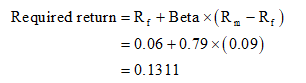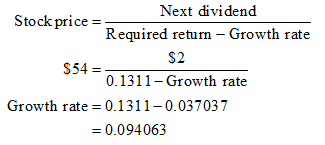# A share of stock with a beta of 0.79 now sells for \$54. Investors expect the stock to pay a year-end dividend of \$2. The T-bill rate is 6%, and the market risk premium is 9%. If the stock is perceived to be fairly priced today, what must be investors’ expectation of the price of the stock at the end of the year? (Do not round intermediate calculations. Round your answer to 2 decimal places.)

Question
24 views

A share of stock with a beta of 0.79 now sells for \$54. Investors expect the stock to pay a year-end dividend of \$2. The T-bill rate is 6%, and the market risk premium is 9%. If the stock is perceived to be fairly priced today, what must be investors’ expectation of the price of the stock at the end of the year? (Do not round intermediate calculations. Round your answer to 2 decimal places.)

check_circle

Step 1

Stock price at the end of the year is \$59.08

For calculating stock price at the end of the year, we need required rate of return and growth rate.

Step 2

Calculation of required return based on CAPM model:Step 3

Calculation of growth rate:Usually, in dividend discount model, next year dividend is used to determine current stock price so the same concept will be used here, to find stock price after one year, dividend after 2 years should be used.

...

### Want to see the full answer?

See Solution

#### Want to see this answer and more?

Solutions are written by subject experts who are available 24/7. Questions are typically answered within 1 hour.*

See Solution
*Response times may vary by subject and question.
Tagged in